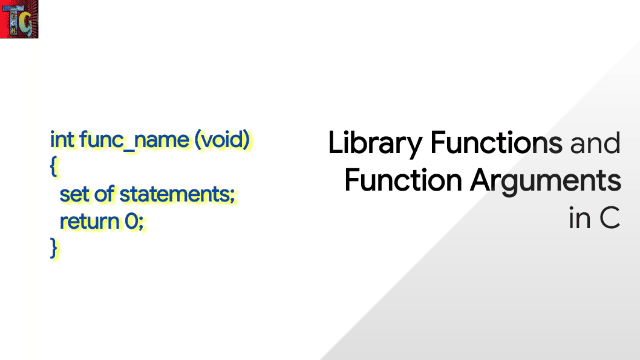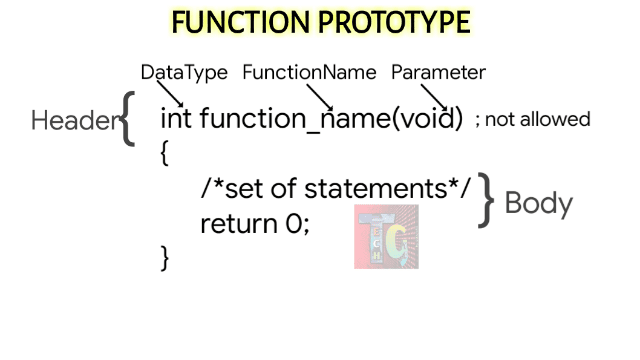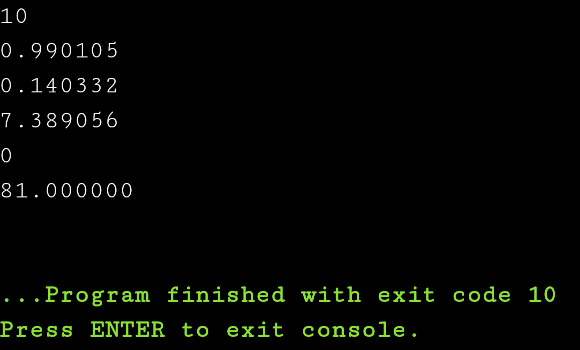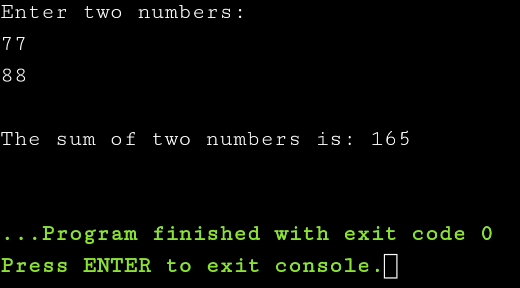# Library Functions and Function Arguments in C

Until now functions are the most familiar stuff that you've all been observing. Yet a detailed study about the functions in C and their types is definitely needed. Let's get started.Library Functions and Function Arguments in C

## What are functions in C?

Any function in C is an enclosed set of statements in a program that when called, executes those statements as per prescribed conditions.

## Advantages of functions in C

There are several advantages of using functions in C. Few of the notable ones are mentioned as under:

• It brings modularity.
• It contributes reusable code.
• In large programs, with the use of functions, debugging and editing becomes simpler.
• Large programs can be combined to smaller portions.

## Declaration of function in C

Whenever any function is declared, it informs compiler about number of parameters accepted by that particular function, data-type of those parameters and return type of that function. Adding parameter names in function declaration is optional, but it is required to add them in definition. I've written down few examples that will clarify:Function Prototype

int test1(int, int);
The above function takes two integers as input and returns one integer value.

int *test2(int*,int);
The above function takes an int pointer and an int variable as input and returns one integer.

char &test3(char x);
The above function takes a character input and returns a reference variable.

int test4(char, int);
The above function takes a character and an integer as input and returns an integer.

## Parts of a function

We can study any function by disintegrating and identifying its main parts:

• Function Prototype
• Function Definition
• Function Call

### Function Prototype:

datatype functionname (parameter list)

In the datatype we mention whether the function will return an integer, float, character etc. type of data.

In functionname we label any name to the function for identifying it throughout the program.

In parameter list we add different types of parameters that a function will take input.

### Function Definition:

``````returntype functionname(function arguments)
{
/*body of the function*/
}``````

The returntype specifies what type of data is to be returned when a function is called.

function arguments contain the datatype and name of variables which are inputted to a function.

### Function Call:

To explain this lets have a look at this program below:

``````#include<stdio.h>
int main()
{
int sum;
printf("The addition of the two numbers is: %d\n",sum);
return 0;
}
{
int i=16,j=9;
return i+j;
}``````

#### Output:Function Calling Output

## Types of functions in C

Broadly functions in C are classified in the following two categories:
• Library Functions: These are built-in functions that come preloaded into library. Thus these are also called Library Functions. e.g. scanf(), printf(), puts(), gets() etc. For using these functions, you need to include the necessary header files in C.
• User Defined Functions: These are functions  defined by the user when the program is written.

C allows to declare and define functions separately, this is specially needed in case of library functions. These library functions are declared in header files and defined in library files. C also lets us both declare and define functions at same place.

However, it is always recommended to declare a function before it is used.

### Library Functions in C

The library functions in C are provided by the system and stored in the library. To use the Library Functions in C, one must add their respective header files, which contain the prototypes and definition of the function.
The following program will demonstrate usage of library functions in C:

``````#include<stdio.h>
#include<ctype.h>
#include<math.h>
void main()
{
int i=-10,j=2,k=10;
float f1=1.43;
double d1=3.0,d2=4.0;
printf("%d\n",abs(i));
printf("%f\n",sin(f1));
printf("%f\n",cos(f1));
printf("%f\n",exp(j));
printf("%d\n",log(k));
printf("%f\n",pow(d1,d2));
}``````

#### Output:Library Function Output in C

### Function Arguments in C

When a function is carried, its arguments can be passed to a function in two ways, call by value and call by reference.

TypeDescription
Call by Value
• The original parameter is passed to a function.
• New memory area is created for the passed parameters, that can be used only within the function.
• The original parameters cannot be modified here.
Call by Reference
• An address is passed to function as parameters, instead of passing it to functions.
• Operator(&) for denoting address is used in the parameter of the called function.
• Changes in function reflect the change of the actual variables.

### Call by value

Here is a sample program which shows a function call by value implementation:

``````#include<stdio.h>
int addition(int num1, int num2);
int main()
{
int sum;
int val1,val2;
printf("Enter two numbers:\n");
scanf("%d%d",&val1,&val2);
printf("\nThe sum of two numbers is: %d\n",sum);
return 0;
}
int addition(int a,int b)
{
return a + b;
}``````

#### Output:Function Call by Value

### Call by reference

Here is a sample program which shows a function call by reference implementation:

``````#include
void swapnum ( int *num1, int *num2 )
{
int temp;
temp = *num1 ;
*num1 = *num2 ;
*num2 = temp ;
}
int main( )
{
int i = 35, j = 45 ;
printf("Before swapping:");
printf("\nnum1 value is %d", i);
printf("\nnum2 value is %d", j);
swapnum( &i, &j );
printf("\nAfter swapping:");
printf("\nnum1 value is %d", i);
printf("\nnum2 value is %d", j);
return 0;
}``````

#### Output:Function Call by Reference

All programs written in this post are compiled online.

### Books I Prefer:I hope this article was helpful to understand types and nature of library functions and function arguments in C! Comment below, if you've got any question. Head back soon for another interesting article on C Programming.